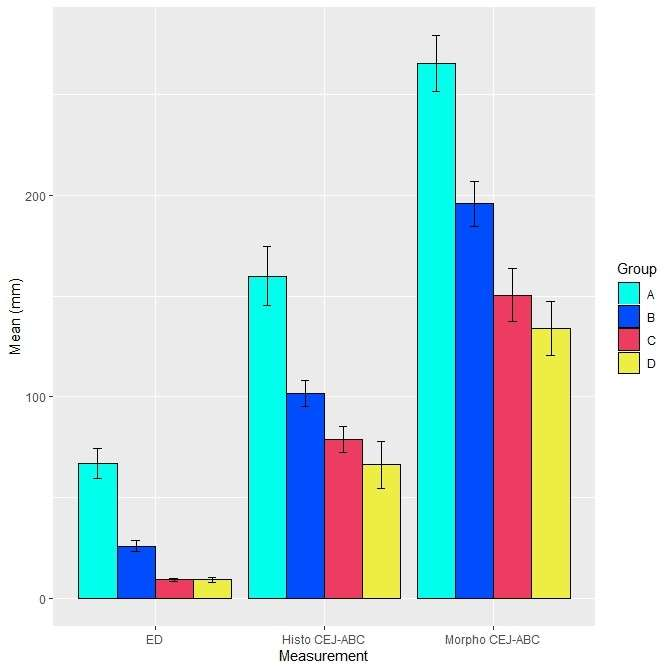# Adding Error Bars to ggplot2 Plots Can be Made Easy Through Dataframe Structure

Adding error bars to plots can be done using different methods (and different error bar calculations) in R. In this blog post, I want to show you what I think is the easiest way to add error bars to ggplot2 plots in R.

First, you need to create your base plot. Both this post and the video demonstrate making the base plot in R. You can see the dataframe used in both cases.

## Adding Error Bar Values to the Plot Dataframe

We are using ggplot2 to make the plot, and what we will end up doing is modifying our base bar plot code by simply adding another line of code to add the object geom_errorbar. What we need to do is to prepare the values for the arguments for geom_errorbar that we will want to set.

The variable named “Mean” in the base plot implies that we might want error bars that represent a certain philosophical margin of error. If we were doing population-based research, we might want this error bar to indicate the 95% confidence interval. If we did that, then we’d want to find the standard deviation of the mean from the original dataset, and multiply that by 1.96 (the z-score) to get the margin of error.

However, in the current use-case which was a lab study, so we decided to use the standard error (SE), which was the standard deviation divided by the square root of the number of units in the group. We decided we would make an error bar spanning 1 SE above the mean to 1 SE below the mean.

The strategy with ggplot2 and geom_errorbar is that when I cam calling geom_errorbar, I need to make sure that each bar in my bar plot has a corresponding SE value available for ggplot2 to use. For me, the easiest way to do that was to create a column in the underlying plot dataframe.

If you access the code files on Github, you’ll see that we are using a data frame called metric_plot_data. If you run the data frame, it will look like this (I included the first two rows):

```> metric_plot_data
Group        Measure       Mean         SE
1      A  Histo CEJ-ABC 159.813741 14.7411291
2      B  Histo CEJ-ABC 101.457411  6.4343916
```

As you can see, I hardcoded the SE in the column titled SE. Now, when I call ggplot2 and refer to geom_errorbar, I can be sure that it puts the right errors on the right bars!

## Code for Adding Error Bars

Now let’s look at our ggplot2 code.

```ggplot(metric_plot_data, aes(x=Measure, y=Mean, fill=Group)) +
geom_bar(position=position_dodge(), stat="identity", color='black') +
ylab("Mean (mm)") +
xlab("Measurement") +
scale_fill_manual(values=cool_colors) +
geom_errorbar(aes(ymin=Mean-SE, ymax=Mean+SE), width=.2, position=position_dodge(.9))
```And here is the plot. Do not be thrown off by scale_fill_manual in the code. This specifies custom colors – you can read more about it here.

I want you to focus on the last line, which is geom_errorbar. Notice in my code that ymin (the minimum y value of the error bar) and ymax (the maximum y value of the error bar) use calculations? Since the mean was a variable, I just made ymin be equal to Mean minus SE, and ymax be Mean plus SE

But if you had unbalanced error bars (e.g., log transformations), you could theoretically hardcode a y-minimum value and call that ymin_value and another ymax_value and put those as two columns in your data. I’m a huge fan of hardcoding as much as you can mathwise in your plot data frame so that you can make sure you do not make a math error when making your plot and calling up equations on-the-fly.

For this plot, the base plot was a bar plot with position=position_dodge(), so we repeat that formatting for the error bars to ensure they land in the right place. The .9 specifies how much of a dodge, and width=.2 specifies the width of the whisker.

Watch this video to learn how to use the ggsave() command to format the dimensions of your plot. That way, you can control the shape of the output, and ensure all your plot features look correct.

Updated October 10, 2022. Added banners June 16, 2023. Added ggsave video August 29, 2023.

Adding error bars to ggplot2 in R plots is easiest if you include the width of the error bar as a variable in your plot data. Read my blog post to see an example.

This site uses Akismet to reduce spam. Learn how your comment data is processed.